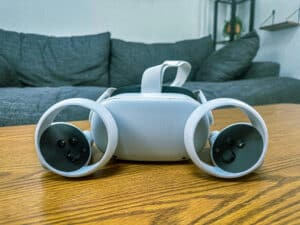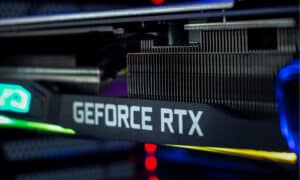You may have experienced this before: you buy a shiny new electric device and excitedly flip through the power ratings manual, only to become stumped by its content. Worry not, you’re not the only one. Even though many of us have a basic understanding of electricity, there may still be a few gray areas we find confusing at times. One such area is the difference between amps and watts.

It’s not unheard of to feel unsure about the distinction between the two, especially when they both relate to the measurement of electricity and power. In this article, we’ll break down the differences between amps and watts in a way that’s easy to understand, and provide some examples to help make sense of it all. So, sit back, and let’s shed some light on the difference between the two.

## Amps vs Watts: What’s the Difference?

Let’s now at look these and other differences in detail.

### Definition

Amps, short for amperes, are the unit of measurement for electric current flow. They represent the amount of electrical charge that passes through a circuit or wire per unit of time.

Just like how a pipe can allow a certain amount of water to flow through it, a wire has a certain capacity for electrical current to flow through it. Amps represent the amount of electric charge passing through that wire per unit of time (seconds).

Watts, on the other hand, are a measure of power. Power is the rate at which energy is transferred or converted, and watts are an expression of this rate.

In the context of electricity, watts represent the amount of energy generated or used by an electrical device or circuit per unit of time, usually in seconds. You can think of watts as the rate at which the water is flowing through the pipe.

It should be noted that besides electricity, watts are also used to measure the power of other forms of energy, such as mechanical and thermal energy since all of them involve the transfer or conversion of energy from one form into another.

### Derivation

#### Current (Amps)

An ampere is a base unit of the International System of Units (SI) with the symbol “A” used for measuring electric current, as we’ve seen. One ampere is defined as the amount of electric charge that passes through a cross-sectional area of a conductor in one second when a current of one coulomb flows through the conductor.

Mathematically, this can be expressed as:

1 A = 1 C/s

where A is the symbol for ampere, C is the symbol for coulomb, and s is the symbol for second.

Suppose a conductor develops a charge of 5 coulombs when an electric current flows through it in 1 second. Then, the current (amps) that would have flowed through the conductor would be:

I = Q/t = 5C / 1s = 5A

#### Power (Watts)

The watt is another derived unit of measurement in the International System of Units (SI), with the symbol “W.” Watts are used for measuring power, which is the rate at which work is done or energy is transferred.

In mathematical terms, power (W) can be expressed using the following equation:

P = E/t

Where P is the power in watts, E is the amount of work done or energy transferred in joules, and t is the time in seconds. One watt is equal to one joule per second (J/s). For example, if a device does 100 joules of work in 5 seconds, the power of the device would be:

P = E/t = 100J / 5s = 20W

Therefore, the power of the device is 20 watts.

### Relationship with Voltage

In addition to understanding the difference between amps and watts from their respective definitions, it is also important to understand how they are related to each other. Amps and watts are related through voltage.

Voltage is the potential difference that enables electric charge to move, and it is measured in volts (V). The relationship between these units can be expressed mathematically as follows:

P = IV

Where P is the power in watts, I is the electric current in amps, and V is the voltage in volts. This formula shows that power is directly proportional to both current and voltage, and is therefore affected by changes in either quantity.

For example, if a device has an electric current of 2 amps and a voltage of 5 volts, the power of the device would be:

P = IV = 2A x 5V = 10W

Therefore, the power of the device is 10 watts.

### Relationship Between Amps and Watts in AC

In an AC circuit, there is a distinction between real power (measured in watts) and apparent power (measured in volt-amperes or VA) due to the presence of reactive components like capacitors and inductors. Real power represents the actual power used in the circuit to perform useful work, such as running an electric motor or lighting a bulb.

For instance, if a 100-watt light bulb is connected to an AC circuit with a voltage of 120 volts and a current of 0.83 amperes, then the real power consumed by the bulb would be 100 watts.

Conversely, apparent power represents the power required to drive the current through the circuit, including any reactive power that is not used to perform useful work.

For example, if a 100-watt load is connected to an AC circuit that has a voltage of 120 volts and a current of 1 ampere, but also has a reactive component like a capacitor or inductor, then the apparent power would be higher than 100 watts due to the presence of the reactive component.

The difference between real power (watts) and apparent power (VA) is known as reactive power and is measured in volt-amperes reactive (VAR). Reactive power is required by reactive components, such as capacitors and inductors, to store and release energy as the AC voltage and current cycle between positive and negative values.

Reactive power does not perform useful work, but it is necessary for the proper operation of some electrical devices and equipment.

## Amps vs Watts: 5 Must-Know Facts

1. The ampere is named after the French physicist André-Marie Ampère, who discovered the relationship between electricity and magnetism in the 1820s.
2. The watt is named after the Scottish engineer James Watt, who made important contributions to the development of steam engines in the late 1700s.
3. Kilowatt-hours (kWh) are the units used by electric companies to measure energy consumption. kWh are determined by multiplying the wattage used by an electrical device over a given period of time by the number of hours it was operated.
4. Lighting fixtures have high wattage (to produce enough light) and low amperage (to reduce heat buildup).
5. Appliances such as refrigerators have both high amperage (to operate their motors) and high wattage ratings (to keep food cool).

## Amps vs Watts: Which One Is Better? Which One Should You Choose?

When it comes to choosing one over the other, it really comes down to the context in which you have to make the choice. If you’re trying to impress your friends with how much sound your new home theater can produce, then watts are definitely the way to go. As we saw, watts are a measure of power, so the higher the number of watts, the more power your sound system can produce.

On the flip side, if you want to know the power consumption of a device or you’re trying to avoid blowing a fuse, then amps are what you need to pay attention to. Amps measure the current flowing through a circuit, and if you exceed the amp rating for your circuit… well, something’s got to give.

You could also consider the case where your electrician is installing a new circuit for a new device you bought; they need to make sure the circuit can handle the amps that will flow through it. Conversely, if you are trying to figure out how much power the new appliance will consume, you need to use watts to determine if it is energy-efficient or not.

It’s therefore important to note that neither amps nor watts are inherently better than the other. They are both useful measurements that serve different purposes, and understanding the difference between them can help you stay safe and protect your appliances — or even save on power bills in the long run.

## Amps vs Watts: What’s the Difference, With Examples FAQs (Frequently Asked Questions)

What’s the relationship between amps and watts?

Their relationship can be explained using the equation Power (P) = Voltage (V) x Current (I). This equation shows that if either one increases or decreases, then so does the other (direct proportion).

For example, if you increase the voltage of a circuit then its current will also increase proportionally. Similarly, if you decrease the voltage then its current will also decrease proportionally.

Is there a device to measure both wattage and amperage?

Yes, there are devices known as wattmeters or power meters that can measure both amps and watts. These devices are used to measure the amount of energy consumed by an electrical device over a given period of time. A multimeter is also capable of measuring current, voltage, and resistance, but it does not have the ability to measure power directly.

Is it dangerous to touch a live wire with low amperage?

Yes, even low amperage can be dangerous if the voltage is high enough. It’s important to always assume that any live wire is dangerous and take appropriate safety precautions.

How do amps and watts affect electricity bills?

Higher wattage devices will result in higher electricity bills than lower wattage devices. Similarly, high amperage circuits will consume more electricity than low amperage circuits since they require more current flow for operation

## More from History-Computer##### Meta Quest 2 vs Oculus Quest 2: Is There a Difference?##### Nvidia RTX 3080 vs 3080-Ti: Full Comparison With Specs, Price, and More##### The 10 Largest Chip Manufacturers in the World and What They Do##### The 10 Largest and Most Important Battery Companies in the World
• The Spruce Available here: https://www.thespruce.com/the-difference-between-watts-vs-volts-4767057
• HowStuffWorks Available here: https://science.howstuffworks.com/environmental/energy/question501.htm
• MrSolar Available here: https://www.mrsolar.com/what-does-volts-amps-ohms-and-watts-mean/
• ElectricDesign Available here: https://www.electronicdesign.com/markets/energy/article/21801657/whats-the-difference-between-watts-and-voltamperes
• The Local Electrician Sydney Available here: https://www.thelocalelectrician.com.au/what-is-the-difference-between-volts-amps-watts/